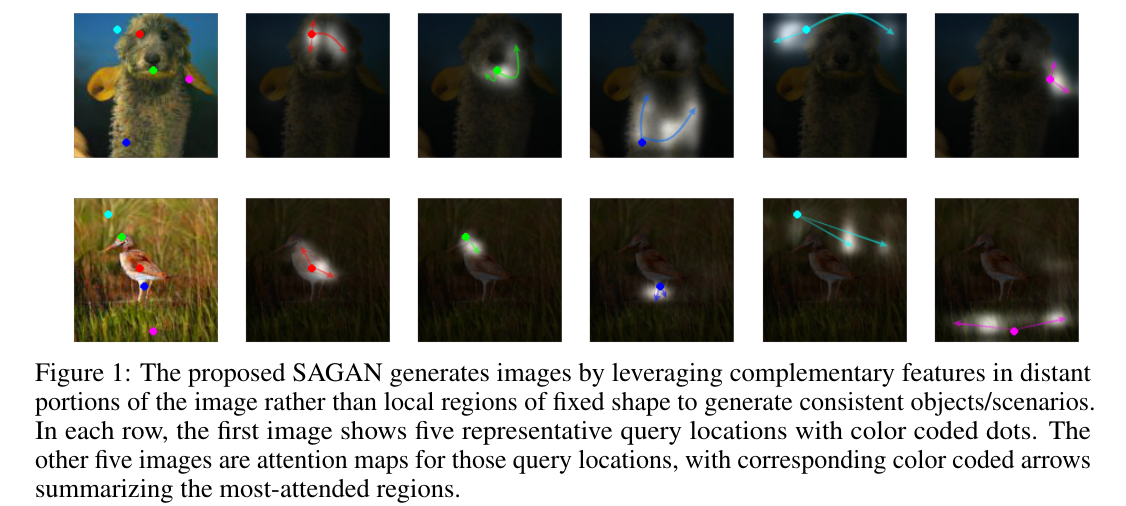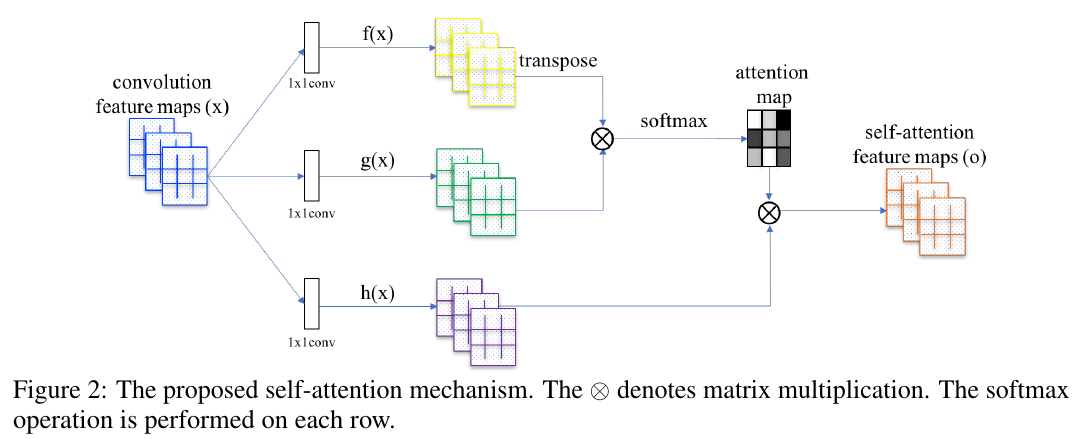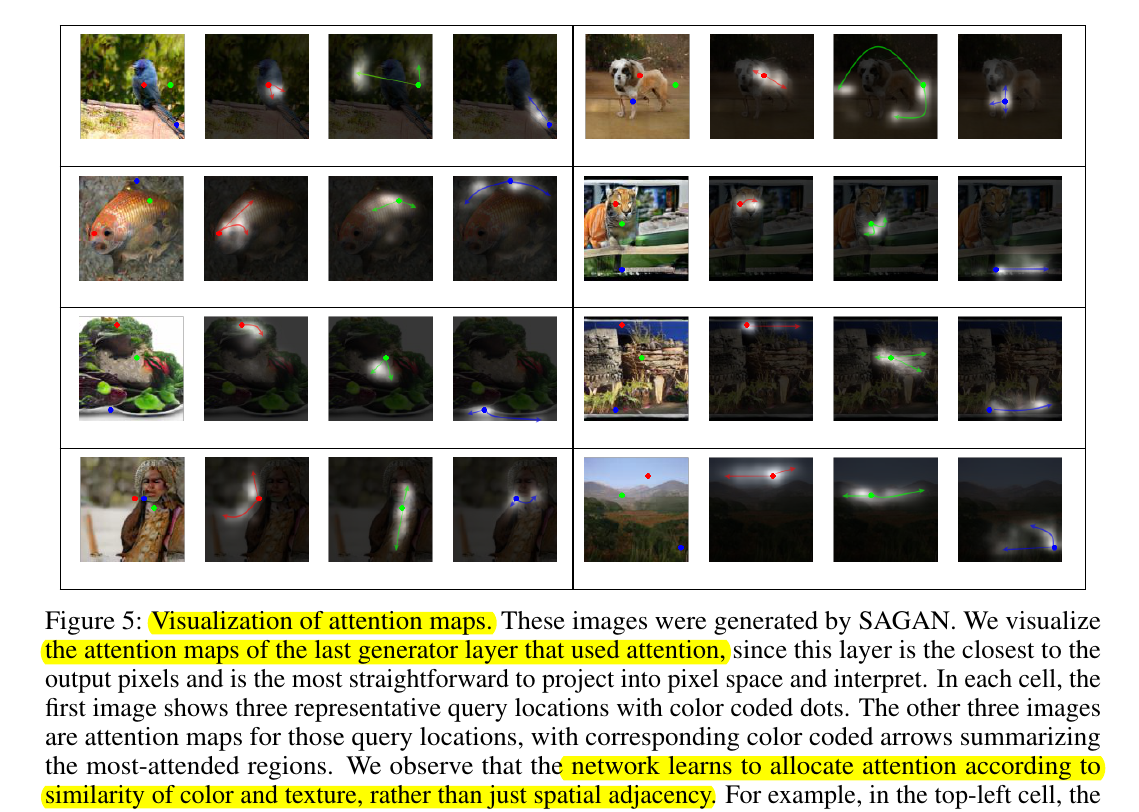### 2. 何方神圣$\begin{cases} & f(x)=W_fx\\ & g(x)=W_gx\\ & h(x)=W_hx \end{cases}$

$\begin{cases} & f(x)\in\mathbb{R}^{\bar{C}*N}\\ & g(x)\in\mathbb{R}^{\bar{C}*N}\\ & h(x)\in\mathbb{R}^{C*N} \end{cases}$

$s_{ij} = f(x_i)^Tg(x_j)$

$\beta_{j,i}=\frac{exp(s_ij)}{\sum_{i=1}^Nexp(s_{ij})}$

$o_j=\sum_{i=1}^N\beta_{j,i}h(x_i)$

$h(x_i)\in\mathbb{R}^{C}$

$o\in\mathbb{R}^{C*N}$

$o$ 即是我们的注意力层了（self-attention feature map）～

$y_i=\gamma o_i + x_i$

$\gamma$ 初始化为 0，训练中更新～～

### 4. 顾盼生姿regards.